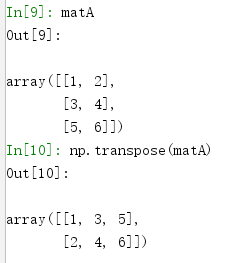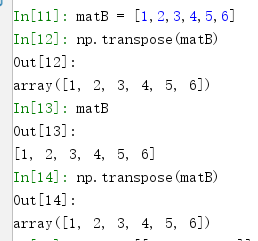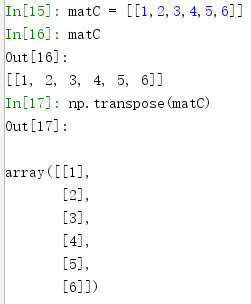• numpy矩阵转置
2020-12-05 16:34:08

Python 2.7.11 (v2.7.11:6d1b6a68f775, Dec  5 2015, 20:32:19) [MSC v.1500 32 bit (

Intel)] on win32

>>> import numpy

>>> a = numpy.mat([[1,2,3,4],[7,8,9,10]])

>>> a

matrix([[ 1,  2,  3,  4],

[ 7,  8,  9, 10]])

>>> b =numpy.transpose(a)

>>> b

matrix([[ 1,  7],

[ 2,  8],

[ 3,  9],

[ 4, 10]])

>>> a.T

matrix([[ 1,  7],

[ 2,  8],

[ 3,  9],

[ 4, 10]])

>>>

更多相关内容
• ## numpy矩阵转置

千次阅读 2022-01-21 13:29:59
numpy 矩阵转置 import numpy as np a1 = np.arange(0,10).reshape(2,5) print(a1) [[0 1 2 3 4] [5 6 7 8 9]] a2 = a1.T print(a2) [[0 5] [1 6] [2 7] [3 8] [4 9]]

# numpy 矩阵转置

import numpy as np

a1 = np.arange(0,10).reshape(2,5)
print(a1)

[[0 1 2 3 4]
[5 6 7 8 9]]

a2 = a1.T
print(a2)

[[0 5]
[1 6]
[2 7]
[3 8]
[4 9]]

展开全文python
• 今天小编就为大家分享一篇Python 使用Numpy矩阵进行转置的方法，具有很好的参考价值，希望对大家有所帮助。一起跟随小编过来看看吧
• ## python numpy矩阵转置

千次阅读 2017-09-29 12:23:48
记录下问题…常规用法如下： 值得注意的是 行向量转换成列向量 或者 列向量转换成行向量，注意矩阵的定义。

记录下问题…

常规用法如下：值得注意的是 行向量转换成列向量 或者 列向量转换成行向量，注意矩阵的定义。

matB中定义使用了[]matC中定义使用了[[]]展开全文python
• 矩阵操作中，经常需要对矩阵进行转置，或者需要交换矩阵的轴，下面介绍一下使用Numpy完成矩阵轴数据交换的几种方法。主要包括以下几种方法： 1、.T转置，适用于1-D，2-D矩阵 2、np.transpose，适用于一次同时...

在矩阵操作中，经常需要对矩阵进行转置，或者需要交换矩阵的轴，下面介绍一下使用Numpy完成矩阵轴数据交换的几种方法。主要包括以下几种方法：

1、.T转置，适用于1-D，2-D矩阵

2、np.transpose，适用于一次同时交换多个（大于两个）轴数据

3、np.swapaxes，一次只能交换两个轴的数据

下面通过一个具体例子感受以下具体的转换过程和转换结果。

import numpy as np

def location(a, v):
"""
在矩阵a中查找元素v的索引编号
:param a:
:param v:
:return:
"""
return [e.tolist() for e in np.where(a == v)]

print("#" * 40, "transpose和swapaxes对比(3维矩阵)", "#" * 40)
x = np.arange(16).reshape(2, 2, 4)
print(x)
print("6在原始矩阵中的索引：", location(x, 6))
# 将矩阵按照新的轴编号进行中心整合
transpose_x = np.transpose(x, axes=[1, 0, 2])
print("6在交换原始矩阵x的0,1轴之后的索引：", location(transpose_x, 6))
# 交换axis1和axis2的轴数据
swapaxes_x = np.swapaxes(x, axis1=0, axis2=1)
print("6在交换原始矩阵x的0,1轴之后的索引：", location(swapaxes_x, 6))
# .T操作相当于np.transpose(x, [2, 1, 0])
T_x = x.T
print("6在T转置之后的索引：", location(T_x, 6))

print("#" * 40, "transpose和swapaxes对比(5维矩阵)", "#" * 40)
x = np.arange(720).reshape(2, 3, 4, 5, 6)
# print(x)
print("100在原始矩阵中的索引：", location(x, 100))
# 变换轴位置
transpose_x = np.transpose(x, axes=[0, 2, 3, 1, 4])
print("100在交换原始矩阵x的[0, 2, 3, 1, 4]轴之后的索引：", location(transpose_x, 100))
# 先交换1 3轴，交换后变成[0, 3, 2, 1, 4]
swapaxes_x_1_3 = np.swapaxes(x, axis1=1, axis2=3)
# 再交换1 2轴，交换后变成[0, 2, 3, 1, 4]
swapaxes_x_2_3 = np.swapaxes(swapaxes_x_1_3, axis1=1, axis2=2)
print("100在交换原始矩阵x的[0, 2, 3, 1, 4]轴之后的索引：", location(swapaxes_x_2_3, 100))
# .T操作相当于np.transpose(x, [4, 3, 2, 1, 0])
T_x = x.T
print("100在T转置之后的索引：", location(T_x, 100))

输出结果如下：

######################################## transpose和swapaxes对比(3维矩阵) ########################################
[[[ 0  1  2  3]
[ 4  5  6  7]]

[[ 8  9 10 11]
[12 13 14 15]]]
6在原始矩阵中的索引： [0, 1, 2]
6在交换原始矩阵x的0,1轴之后的索引： [1, 0, 2]
6在交换原始矩阵x的0,1轴之后的索引： [1, 0, 2]
6在T转置之后的索引： [2, 1, 0]
######################################## transpose和swapaxes对比(5维矩阵) ########################################
100在原始矩阵中的索引： [0, 0, 3, 1, 4]
100在交换原始矩阵x的[0, 2, 3, 1, 4]轴之后的索引： [0, 3, 1, 0, 4]
100在交换原始矩阵x的[0, 2, 3, 1, 4]轴之后的索引： [0, 3, 1, 0, 4]
100在T转置之后的索引： [4, 1, 3, 0, 0]

总结一下：

1、.T矩阵转置就相当于将矩阵的轴从0-n变成了n-0

2、transpose方法就是按照传入的轴编号顺序进行轴数据交换

3、swapaxes方法就是将传入的两个轴数据进行交换

展开全文python transpose swapaxes
• numpy矩阵转置The transpose of a matrix is obtained by moving the rows data to the column and columns data to the rows. 通过将行数据移动到列，将列数据移动到行，可以获得矩阵转置。 If we have an ...列表 python matlab 机器学习
• 矩阵乘法1.使用运算符 *2.使用numpy.multiply()方法3.使用numpy.dot()方法三.一维数组的特例 一.转置 数组转置 1.使用 array.T: 1.对于array对象，若是一维数组（行向量），array.T并不会把行向量转化成列向量，...python
• 进行矩阵运算时，经常要用数组转置，比如计算矩阵内积X^T X.这时就需要利用数组转置，如下： 2.轴对换之transpose 对于高维数组，可以使用轴对换来对多个维度进行变换。 这里创建了一个三维数组，各维度大小...
• Numpy numpy.transpose(),可以实现矩阵转置。 也可以输入参数，实现行与列的转置 # making a 3x3 array a = np.array([[1, 2], [4, 5], [7, 8]]) # before transpose print(a, end ='\n\n') # after ...
• ## 5、数组（矩阵转置和轴对换： 1、numpy中的数组其实就是线性代数中的矩阵矩阵是可以进行转置的。ndarray有一个T属性，可以返回这个数组的转置的结果。示例代码如下： `python #ndarray有一个T属性，可以...python
• 天下难事，必作于易；天下大事，必作于细——老子 ...进行矩阵运算时，经常要用数组转置，比如计算矩阵内积X^T X.这时就需要利用数组转置，如下： 2.轴对换之transpose 对于高维数组，可以使用轴对换来对多...transpose swapaxes
• 主要介绍了Python实现的矩阵转置与矩阵相乘运算,结合实例形式分析了Python针对矩阵进行转置与相乘运算的相关实现技巧与操作注意事项,需要的朋友可以参考下
• 转置 首先确定python里有numpy这个模块。 import numpy as np arr=np.arange(9).reshape(3,3) #转置 arr.T #矩阵相乘 np.matmul(arr.T,arr)python
• 最近看代码的时候，老是出现np.transpose()这个用法，但是对其中的原理还是不甚了解，今天就来总结一下，以及这个用法对...返回值 p:ndarray 返回转置过后的原数组的视图。给大家举个例子。对于一维数组：>>&...
• 利用Python进行数据分析时，Numpy是最常用的库，经常用来对数组、矩阵等进行转置等，有时候用来做数据的存储。 在numpy中，转置transpose和轴对换是很基本的操作，下面分别详细讲述一下，以免自己忘记。 In : ...
• import numpy as np a = np.array([[-2, 3, 3], [1, -1, 0], [-1, 2, 1]]) b =np.linalg.inv(a) c = np.array([[-1, -1, 1], [3, 1, 1], [3, 3, -1]]) d ...
• [Python]使用Numpy矩阵进行转置    matrix.py   #!/usr/bin/python # -*- encoding:UTF-8-*- import pprint import numpy as np matrix = [[1,2],[3,4],[5,6]] print('列表：') pprint.pprint(matrix) ...
• 矩阵转置 >>> import numpy as np >>> arr=np.arange(15).reshape((3,5)) >>> arr array([[ 0, 1, 2, 3, 4], [ 5, 6, 7, 8, 9], [10, 11, 12, 13, 14]]) >>> arr.T array([[ 0, 5, 10], [ 1, 6, 11], [ 2, 7...
• ##Numpy 一位矩阵转置问题 numpy中，我们如果直接对一维行矩阵转置，会出现问题 这时，可以采用expand_dims import numpy as np d=np.zeros((1,9)) d.shape d=np.expand_dims(d,axis=0) d.shape d.T d.transpose() ...机器学习
• Numpy中两大重要类型array类型(N维数组ndarray)和矩阵类型matrix是很多基于Numpy数值计算的基础，因此搞清楚两者的关系是相当重要的， 特别是程序中混杂了这两种类型，还夹带着一些加减乘除的运算，那简直有一种剪...
• #转置 t=np.arange(27).reshape(3,3,3) #适合低维数组(二维以内) t.T # t.transpose() t.swapaxes(1,0)
• import numpy as np &gt;&gt;&gt; t=np.arange(4) # 插入值0-3 &gt;&gt;&gt; t array([0, 1, 2, 3]) &gt;&gt;&gt; t.transpose() array([0, 1, 2, 3]) &gt;&gt;&...
• demo.py（numpy数组转置的3种方法）： # coding=utf-8 import numpy as np t1 = np.arange(24).reshape(...# 方法一：矩阵转置 transpose() t2 = t1.transpose() print(t2) # 6行4列的二维数组 # 方法二：...Python
• import numpy as np #矩阵创建 matrix1 = np.mat(“1 2 3;4 5 6;7 8 9”) print(matrix1,type(matrix1)) matrix2 = np.matrix([[1,2,3],[4,5,6],[7,8,9]]) print(matrix2) arr1 = np.eye(3) arr2 = 3*np.eye(3) ......